# mscohere

Magnitude-squared coherence

## Syntax

``cxy = mscohere(x,y)``
``cxy = mscohere(x,y,window)``
``cxy = mscohere(x,y,window,noverlap)``
``cxy = mscohere(x,y,window,noverlap,nfft)``
``cxy = mscohere(___,'mimo')``
``[cxy,w] = mscohere(___)``
``[cxy,f] = mscohere(___,fs)``
``[cxy,w] = mscohere(x,y,window,noverlap,w)``
``[cxy,f] = mscohere(x,y,window,noverlap,f,fs)``
``[___] = mscohere(x,y,___,freqrange)``
``mscohere(___)``

## Description

````cxy = mscohere(x,y)` finds the magnitude-squared coherence estimate, `cxy`, of the input signals, `x` and `y`. If `x` and `y` are both vectors, they must have the same length.If one of the signals is a matrix and the other is a vector, then the length of the vector must equal the number of rows in the matrix. The function expands the vector and returns a matrix of column-by-column magnitude-squared coherence estimates.If `x` and `y` are matrices with the same number of rows but different numbers of columns, then `mscohere` returns a multiple coherence matrix. The mth column of `cxy` contains an estimate of the degree of correlation between all the input signals and the mth output signal. See Magnitude-Squared Coherence for more information.If `x` and `y` are matrices of equal size, then `mscohere` operates column-wise: `cxy(:,n) = mscohere(x(:,n),y(:,n))`. To obtain a multiple coherence matrix, append `'mimo'` to the argument list. ```
````cxy = mscohere(x,y,window)` uses `window` to divide `x` and `y` into segments and perform windowing. You must use at least two segments. Otherwise, the magnitude-squared coherence is 1 for all frequencies. In the MIMO case, the number of segments must be greater than the number of input channels.```
````cxy = mscohere(x,y,window,noverlap)` uses `noverlap` samples of overlap between adjoining segments.```

example

````cxy = mscohere(x,y,window,noverlap,nfft)` uses `nfft` sampling points to calculate the discrete Fourier transform.```

example

````cxy = mscohere(___,'mimo')` computes a multiple coherence matrix for matrix inputs. This syntax can include any combination of input arguments from previous syntaxes.```
````[cxy,w] = mscohere(___)` returns a vector of normalized frequencies, `w`, at which the magnitude-squared coherence is estimated.```

example

````[cxy,f] = mscohere(___,fs)` returns a vector of frequencies, `f`, expressed in terms of the sample rate, `fs`, at which the magnitude-squared coherence is estimated. `fs` must be the sixth numeric input to `mscohere`. To input a sample rate and still use the default values of the preceding optional arguments, specify these arguments as empty, `[]`.```
````[cxy,w] = mscohere(x,y,window,noverlap,w)` returns the magnitude-squared coherence estimate at the normalized frequencies specified in `w`.```
````[cxy,f] = mscohere(x,y,window,noverlap,f,fs)` returns the magnitude-squared coherence estimate at the frequencies specified in `f`.```
````[___] = mscohere(x,y,___,freqrange)` returns the magnitude-squared coherence estimate over the frequency range specified by `freqrange`. Valid options for `freqrange` are `'onesided'`, `'twosided'`, and `'centered'`.```

example

````mscohere(___)` with no output arguments plots the magnitude-squared coherence estimate in the current figure window.```

## Examples

collapse all

Compute and plot the coherence estimate between two colored noise sequences.

Generate a signal consisting of white Gaussian noise.

`r = randn(16384,1);`

To create the first sequence, bandpass filter the signal. Design a 16th-order filter that passes normalized frequencies between 0.2π and 0.4π rad/sample. Specify a stopband attenuation of 60 dB. Filter the original signal.

```dx = designfilt('bandpassiir','FilterOrder',16, ... 'StopbandFrequency1',0.2,'StopbandFrequency2',0.4, ... 'StopbandAttenuation',60); x = filter(dx,r);```

To create the second sequence, design a 16th-order filter that stops normalized frequencies between 0.6π and 0.8π rad/sample. Specify a passband ripple of 0.1 dB. Filter the original signal.

```dy = designfilt('bandstopiir','FilterOrder',16, ... 'PassbandFrequency1',0.6,'PassbandFrequency2',0.8, ... 'PassbandRipple',0.1); y = filter(dy,r);```

Estimate the magnitude-squared coherence of `x` and `y`. Use a 512-sample Hamming window. Specify 500 samples of overlap between adjoining segments and 2048 DFT points.

`[cxy,fc] = mscohere(x,y,hamming(512),500,2048);`

Plot the coherence function and overlay the frequency responses of the filters.

```[qx,f] = freqz(dx); qy = freqz(dy); plot(fc/pi,cxy) hold on plot(f/pi,abs(qx),f/pi,abs(qy)) hold off```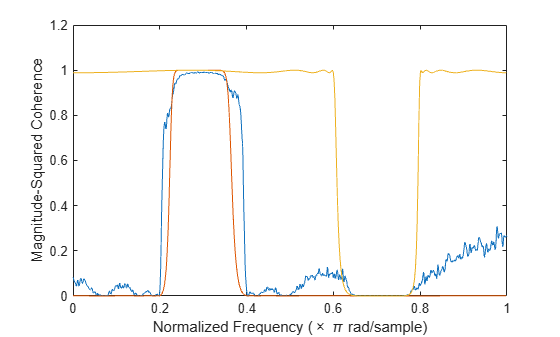Generate a random two-channel signal, `x`. Generate another signal, `y`, by lowpass filtering the two channels and adding them together. Specify a 30th-order FIR filter with a cutoff frequency of 0.3π and designed using a rectangular window.

```h = fir1(30,0.3,rectwin(31)); x = randn(16384,2); y = sum(filter(h,1,x),2);```

Compute the multiple-coherence estimate of `x` and `y`. Window the signals with a 1024-sample Hann window. Specify 512 samples of overlap between adjoining segments and 1024 DFT points. Plot the estimate.

```noverlap = 512; nfft = 1024; mscohere(x,y,hann(nfft),noverlap,nfft,'mimo')```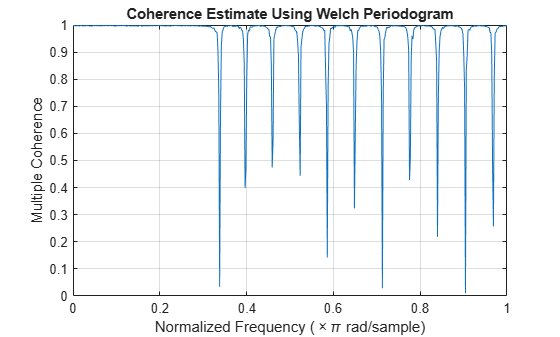Compare the coherence estimate to the frequency response of the filter. The drops in coherence correspond to the zeros of the frequency response.

```[H,f] = freqz(h); hold on yyaxis right plot(f/pi,20*log10(abs(H))) hold off```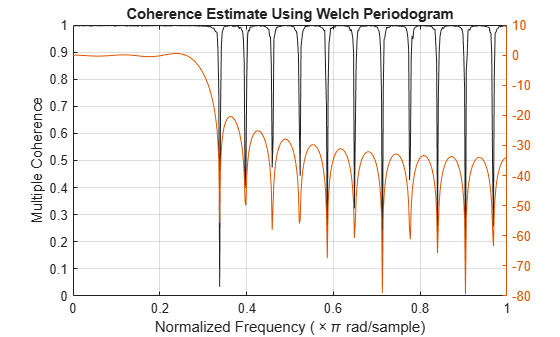Compute and plot the ordinary magnitude-squared coherence estimate of `x` and `y`. The estimate does not reach 1 for any of the channels.

```figure mscohere(x,y,hann(nfft),noverlap,nfft)```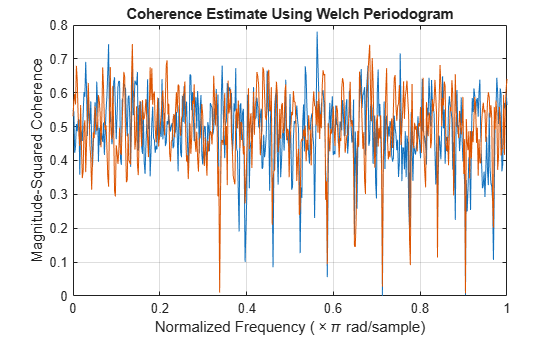Generate two multichannel signals, each sampled at 1 kHz for 2 seconds. The first signal, the input, consists of three sinusoids with frequencies of 120 Hz, 360 Hz, and 480 Hz. The second signal, the output, is composed of two sinusoids with frequencies of 120 Hz and 360 Hz. One of the sinusoids lags the first signal by π/2. The other sinusoid has a lag of π/4. Both signals are embedded in white Gaussian noise.

```fs = 1000; f = 120; t = (0:1/fs:2-1/fs)'; inpt = sin(2*pi*f*[1 3 4].*t); inpt = inpt+randn(size(inpt)); oupt = sin(2*pi*f*[1 3].*t-[pi/2 pi/4]); oupt = oupt+randn(size(oupt));```

Estimate the degree of correlation between all the input signals and each of the output channels. Use a Hamming window of length 100 to window the data. `mscohere` returns one coherence function for each output channel. The coherence functions reach maxima at the frequencies shared by the input and the output.

```[Cxy,f] = mscohere(inpt,oupt,hamming(100),[],[],fs,'mimo'); for k = 1:size(oupt,2) subplot(size(oupt,2),1,k) plot(f,Cxy(:,k)) title(['Output ' int2str(k) ', All Inputs']) end```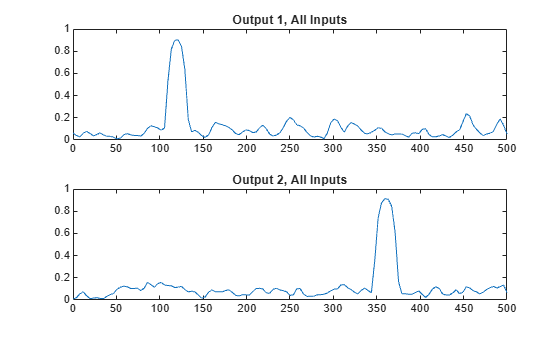Switch the input and output signals and compute the multiple coherence function. Use the same Hamming window. There is no correlation between input and output at 480 Hz. Thus there are no peaks in the third correlation function.

```[Cxy,f] = mscohere(oupt,inpt,hamming(100),[],[],fs,'mimo'); for k = 1:size(inpt,2) subplot(size(inpt,2),1,k) plot(f,Cxy(:,k)) title(['Input ' int2str(k) ', All Outputs']) end```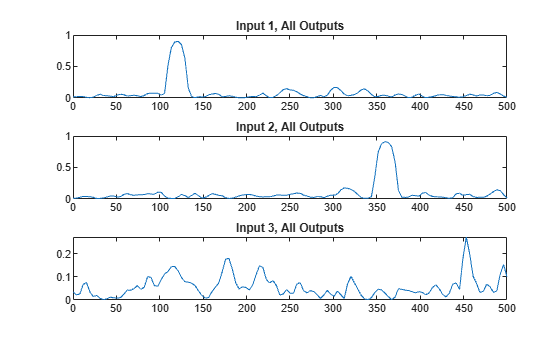Repeat the computation, using the plotting functionality of `mscohere`.

```clf mscohere(oupt,inpt,hamming(100),[],[],fs,'mimo')```Compute the ordinary coherence function of the second signal and the first two channels of the first signal. The off-peak values differ from the multiple coherence function.

```[Cxy,f] = mscohere(oupt,inpt(:,[1 2]),hamming(100),[],[],fs); plot(f,Cxy)```Find the phase differences by computing the angle of the cross-spectrum at the points of maximum coherence.

```Pxy = cpsd(oupt,inpt(:,[1 2]),hamming(100),[],[],fs); [~,mxx] = max(Cxy); for k = 1:2 fprintf('Phase lag %d = %5.2f*pi\n',k,angle(Pxy(mxx(k),k))/pi) end```
```Phase lag 1 = -0.51*pi Phase lag 2 = -0.22*pi ```

Generate two sinusoidal signals sampled for 1 second each at 1 kHz. Each sinusoid has a frequency of 250 Hz. One of the signals lags the other in phase by π/3 radians. Embed both signals in white Gaussian noise of unit variance.

```fs = 1000; f = 250; t = 0:1/fs:1-1/fs; um = sin(2*pi*f*t)+rand(size(t)); un = sin(2*pi*f*t-pi/3)+rand(size(t));```

Use `mscohere` to compute and plot the magnitude-squared coherence of the signals.

`mscohere(um,un,[],[],[],fs)`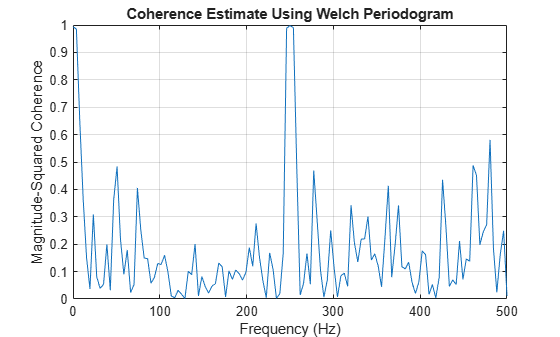Modify the title of the plot, the label of the x-axis, and the limits of the y-axis.

```title('Magnitude-Squared Coherence') xlabel('f (Hz)') ylim([0 1.1])```Use `gca` to obtain a handle to the current axes. Change the locations of the tick marks. Remove the label of the y-axis.

```ax = gca; ax.XTick = 0:250:500; ax.YTick = 0:0.25:1; ax.YLabel.String = [];```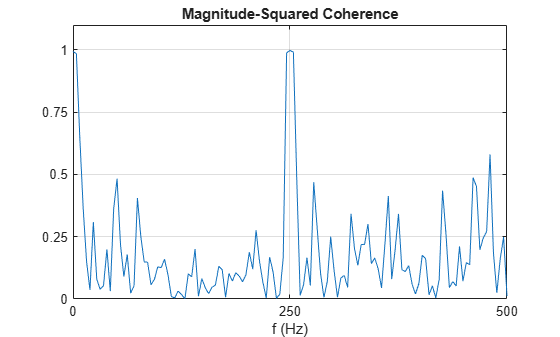Call the `Children` property of the handle to change the color and width of the plotted line.

```ln = ax.Children; ln.Color = [0.8 0 0]; ln.LineWidth = 1.5;```Alternatively, use `set` and `get` to modify the line properties.

`set(get(gca,'Children'),'Color',[0 0.4 0],'LineStyle','--','LineWidth',1)`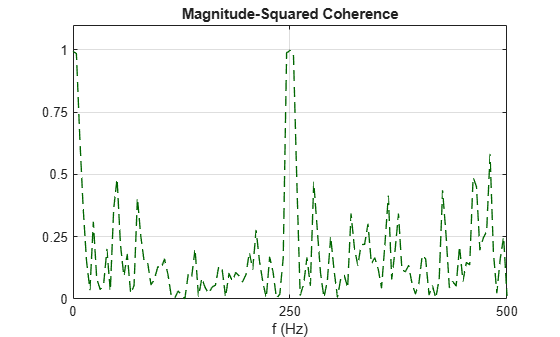## Input Arguments

collapse all

Input signals, specified as vectors or matrices.

Example: `cos(pi/4*(0:159))+randn(1,160)` specifies a sinusoid embedded in white Gaussian noise.

Data Types: `single` | `double`
Complex Number Support: Yes

Window, specified as an integer or as a row or column vector. Use `window` to divide the signal into segments:

• If `window` is an integer, then `mscohere` divides `x` and `y` into segments of length `window` and windows each segment with a Hamming window of that length.

• If `window` is a vector, then `mscohere` divides `x` and `y` into segments of the same length as the vector and windows each segment using `window`.

If the length of `x` and `y` cannot be divided exactly into an integer number of segments with `noverlap` overlapping samples, then the signals are truncated accordingly.

If you specify `window` as empty, then `mscohere` uses a Hamming window such that `x` and `y` are divided into eight segments with `noverlap` overlapping samples.

For a list of available windows, see Windows.

Example: `hann(N+1)` and `(1-cos(2*pi*(0:N)'/N))/2` both specify a Hann window of length `N` + 1.

Data Types: `single` | `double`

Number of overlapped samples, specified as a positive integer.

• If `window` is scalar, then `noverlap` must be smaller than `window`.

• If `window` is a vector, then `noverlap` must be smaller than the length of `window`.

If you specify `noverlap` as empty, then `mscohere` uses a number that produces 50% overlap between segments. If the segment length is unspecified, the function sets `noverlap` to ⌊N/4.5⌋, where N is the length of the input and output signals.

Data Types: `double` | `single`

Number of DFT points, specified as a positive integer. If you specify `nfft` as empty, then `mscohere` sets this argument to max(256,2p), where p = ⌈log2 N for input signals of length N.

Data Types: `single` | `double`

Sample rate, specified as a positive scalar. The sample rate is the number of samples per unit time. If the unit of time is seconds, then the sample rate has units of Hz.

Normalized frequencies, specified as a row or column vector with at least two elements. Normalized frequencies are in rad/sample.

Example: `w = [pi/4 pi/2]`

Data Types: `double`

Frequencies, specified as a row or column vector with at least two elements. The frequencies are in cycles per unit time. The unit time is specified by the sample rate, `fs`. If `fs` has units of samples/second, then `f` has units of Hz.

Example: `fs = 1000; f = [100 200]`

Data Types: `double`

Frequency range for the magnitude-squared coherence estimate, specified as `'onesided'`, `'twosided'`, or `'centered'`. The default is `'onesided'` for real-valued signals and `'twosided'` for complex-valued signals.

• `'onesided'` — Returns the one-sided estimate of the magnitude-squared coherence estimate between two real-valued input signals, `x` and `y`. If `nfft` is even, `cxy` has `nfft`/2 + 1 rows and is computed over the interval [0,π] rad/sample. If `nfft` is odd, `cxy` has (`nfft` + 1)/2 rows and the interval is [0,π) rad/sample. If you specify `fs`, the corresponding intervals are [0,`fs`/2] cycles/unit time for even `nfft` and [0,`fs`/2) cycles/unit time for odd `nfft`.

• `'twosided'` — Returns the two-sided estimate of the magnitude-squared coherence estimate between two real-valued or complex-valued input signals, `x` and `y`. In this case, `cxy` has `nfft` rows and is computed over the interval [0,2π) rad/sample. If you specify `fs`, the interval is [0,`fs`) cycles/unit time.

• `'centered'` — Returns the centered two-sided estimate of the magnitude-squared coherence estimate between two real-valued or complex-valued input signals, `x` and `y`. In this case, `cxy` has `nfft` rows and is computed over the interval (–π,π] rad/sample for even `nfft` and (–π,π) rad/sample for odd `nfft`. If you specify `fs`, the corresponding intervals are (–`fs`/2, `fs`/2] cycles/unit time for even `nfft` and (–`fs`/2, `fs`/2) cycles/unit time for odd `nfft`.

## Output Arguments

collapse all

Magnitude-squared coherence estimate, returned as a vector, matrix, or three-dimensional array.

Normalized frequencies, returned as a real-valued column vector.

Frequencies, returned as a real-valued column vector.

collapse all

### Magnitude-Squared Coherence

The magnitude-squared coherence estimate is a function of frequency with values between 0 and 1. These values indicate how well `x` corresponds to `y` at each frequency. The magnitude-squared coherence is a function of the power spectral densities, Pxx(f) and Pyy(f), and the cross power spectral density, Pxy(f), of `x` and `y`:

`${C}_{xy}\left(f\right)=\frac{{|{P}_{xy}\left(f\right)|}^{2}}{{P}_{xx}\left(f\right){P}_{yy}\left(f\right)}.$`

For multi-input/multi-output systems, the multiple-coherence function becomes

`$\begin{array}{c}{C}_{X{y}_{i}}\left(f\right)=\frac{{P}_{X{y}_{i}}^{†}\left(f\right){P}_{XX}^{-1}\left(f\right){P}_{X{y}_{i}}\left(f\right)}{{P}_{{y}_{i}{y}_{i}}\left(f\right)}\\ =\left[\begin{array}{ccc}{P}_{{x}_{1}{y}_{i}}^{*}\left(f\right)& \cdots & {P}_{{x}_{m}{y}_{i}}^{*}\left(f\right)\end{array}\right]{\left[\begin{array}{cccc}{P}_{{x}_{1}{x}_{1}}\left(f\right)& {P}_{{x}_{1}{x}_{2}}\left(f\right)& \cdots & {P}_{{x}_{1}{x}_{m}}\left(f\right)\\ {P}_{{x}_{2}{x}_{1}}\left(f\right)& {P}_{{x}_{2}{x}_{2}}\left(f\right)& \cdots & {P}_{{x}_{2}{x}_{m}}\left(f\right)\\ ⋮& ⋮& \ddots & ⋮\\ {P}_{{x}_{m}{x}_{1}}\left(f\right)& {P}_{{x}_{m}{x}_{2}}\left(f\right)& \cdots & {P}_{{x}_{m}{x}_{m}}\left(f\right)\end{array}\right]}^{-1}\left[\begin{array}{c}{P}_{{x}_{1}{y}_{i}}\left(f\right)\\ ⋮\\ {P}_{{x}_{m}{y}_{i}}\left(f\right)\end{array}\right]\frac{1}{{P}_{{y}_{i}{y}_{i}}\left(f\right)}\end{array}$`

for the ith output signal, where:

• X corresponds to the array of m inputs.

• PXyi is the m-dimensional vector of cross power spectral densities between the inputs and yi.

• PXX is the m-by-m matrix of power spectral densities and cross power spectral densities of the inputs.

• Pyiyi is the power spectral density of the output.

• The dagger (†) stands for the complex conjugate transpose.

## Algorithms

`mscohere` estimates the magnitude-squared coherence function  using Welch’s overlapped averaged periodogram method , .

 Gómez González, A., J. Rodríguez, X. Sagartzazu, A. Schumacher, and I. Isasa. “Multiple Coherence Method in Time Domain for the Analysis of the Transmission Paths of Noise and Vibrations with Non-Stationary Signals.” Proceedings of the 2010 International Conference of Noise and Vibration Engineering, ISMA2010-USD2010. pp. 3927–3941.

 Kay, Steven M. Modern Spectral Estimation. Englewood Cliffs, NJ: Prentice-Hall, 1988.

 Rabiner, Lawrence R., and Bernard Gold. Theory and Application of Digital Signal Processing. Englewood Cliffs, NJ: Prentice-Hall, 1975.

 Stoica, Petre, and Randolph Moses. Spectral Analysis of Signals. Upper Saddle River, NJ: Prentice Hall, 2005.

 Welch, Peter D. “The Use of Fast Fourier Transform for the Estimation of Power Spectra: A Method Based on Time Averaging Over Short, Modified Periodograms.” IEEE® Transactions on Audio and Electroacoustics. Vol. AU-15, 1967, pp. 70–73.